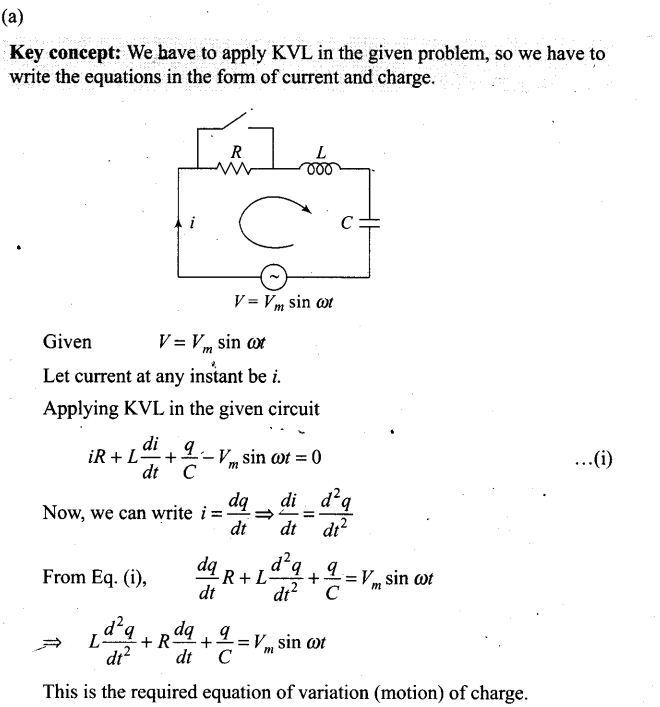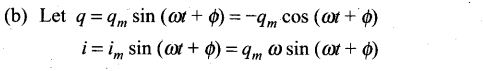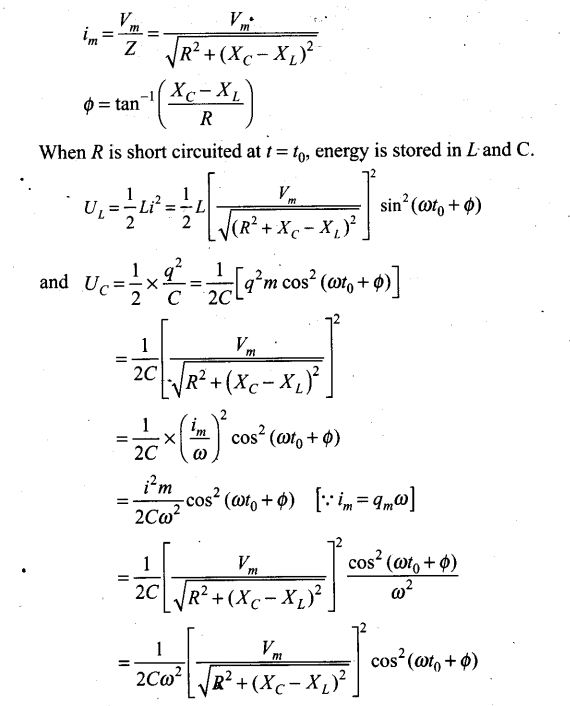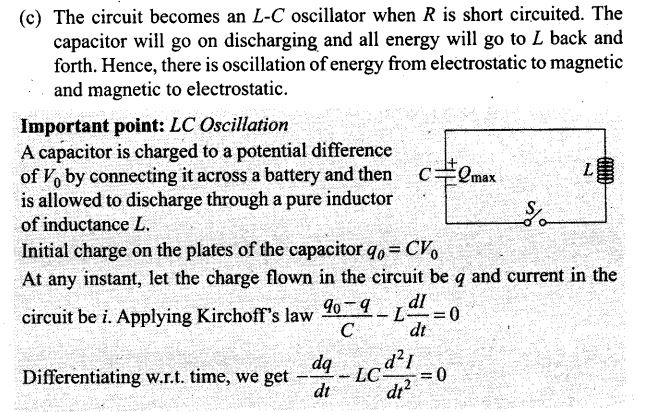# Alternating Current NCERT Exemplar Problems Solutions Physics

0
1840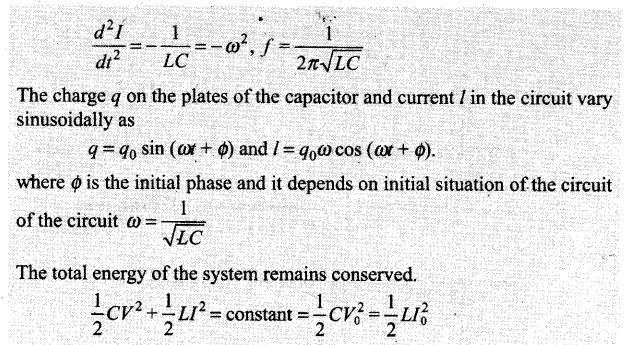## NCERT Exemplar Problems Class 12 Physics Chapter 7 Alternating Current

Multiple Choice Questions (MCQs)
Question 1. If the rms current in a 50 Hz AC circuit is 5 A, the value of the current 1/300 s after its value becomes zero is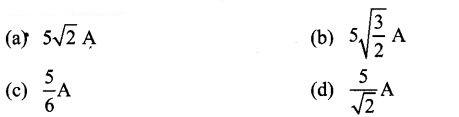Solution: (b)
Key concept: Equation for i and V: Alternating current or voltage varying as sine function can be written as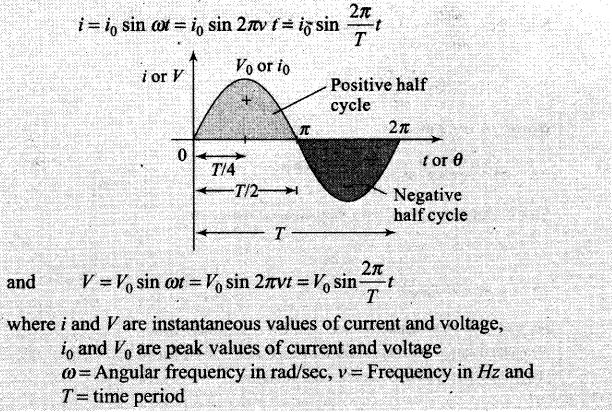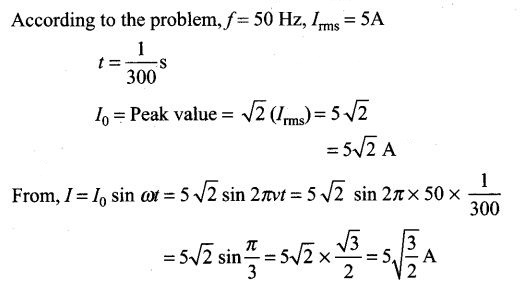Question 2. An alternating current generator has an internal resistance Rgand an internal reactance Xg It is used to supply power to a passive load consisting of a resistance Rg and a reactance XL. For maximum power to be delivered from the generator to the load, the value of XL is equal to
(a) zero (b) Xg
(c) -Xg (d) Rg
Solution: (c) For maximum power to be delivered from the generator (or internal reactance Xg) to the load (of reactance, XL),
=> XL + Xg = 0 (the total reactance must vanish)
=>XL=-Xg

Question 3.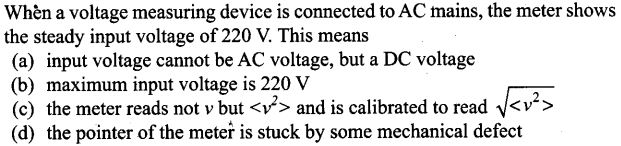Solution: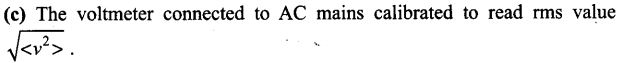Question 4. To reduce the resonant frequency in an L-C-R series circuit with a generator,
(a) the generator frequency should be reduced
(b) another capacitor should be added in parallel to the first
(c) the iron core of the inductor should be removed
(d) dielectric in the capacitor should be removed
Solution: (b)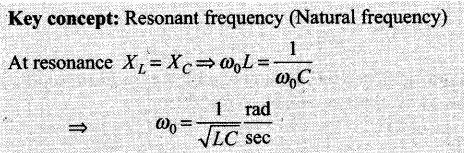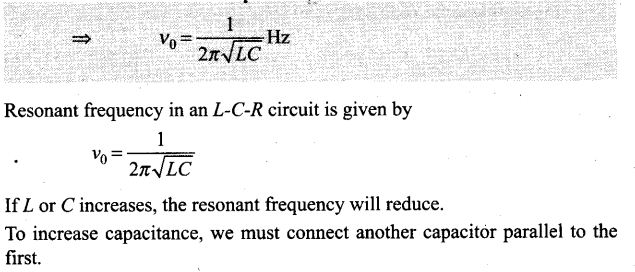Question 5. Which of the following combinations should be selected for better tuning of an L-C-R circuit used for communication?
(a) R = 20 Ω, L = 1.5 H, C = 35μF
(b) R = 25 Ω, L = 2.5 H, C = 45 μF
(c) R=15Ω, L = 3.5H, C = 30 μF
(d) R = 25 Ω, L = 1.5 H, C = 45 μF
Solution: (c)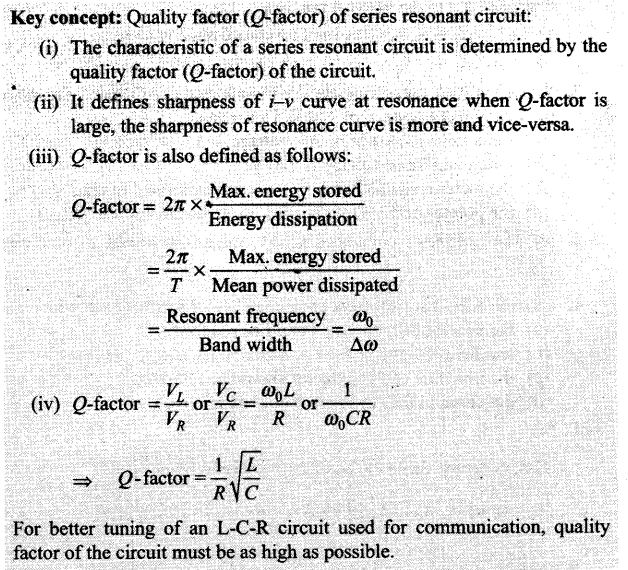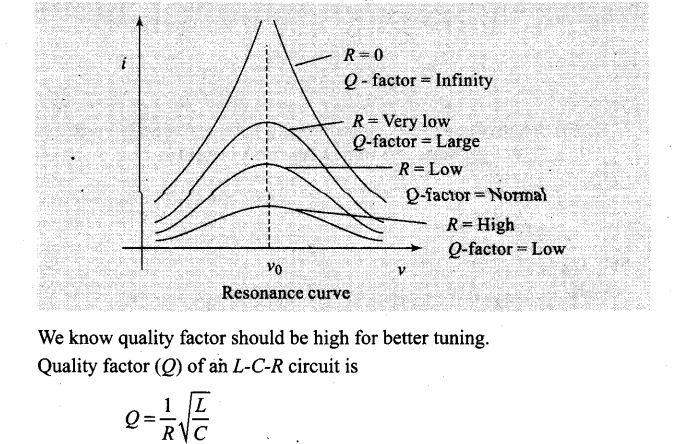where R is the resistance, L is the inductance and C is the capacitance of the circuit.
For high Q factor R should be low, L should be high and C should be low. These conditions are best satisfied by the values given in option (c).
Important point: Be careful while writing formula for quality factor, this formula we used in this case is only for series L-C-R circuit.

Question 6. An inductor of reactance 1 Ω and a resistor of 2 Ω are connected in series to the terminals of a 6 V (rms) AC source. The power dissipated in the circuit is
(a) 8 W (b) 12 W
(c) 14.4 W (d) 18 W
Solution: (c) According to the problem, XL = 1 Ω , R = 2 Ω ,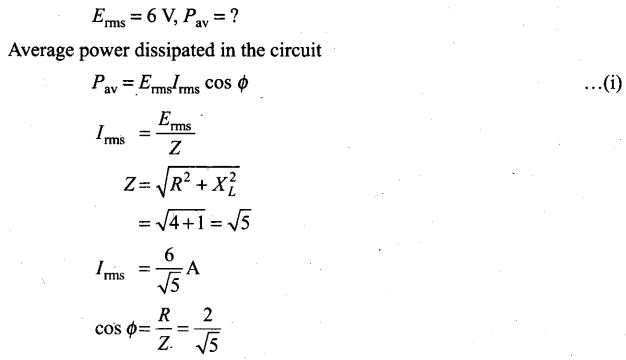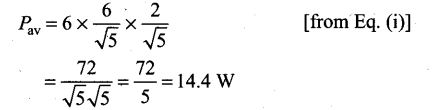Question 7. The output of a step-down transformer is measured to be 24 V when connected to a 12 W light bulb. The value of the peak current is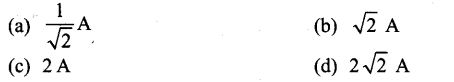Solution: (a)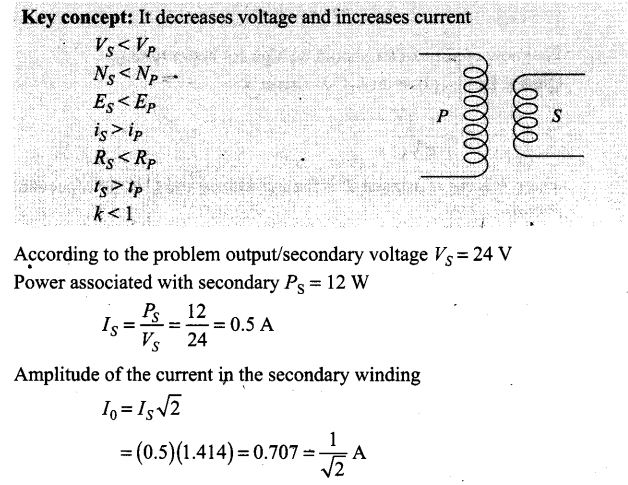One or More Than One Correct Answer Type
Question 8. As the frequency of an AC circuit increases, the current first increases and then decreases. What combination of circuit elements is most likely to comprise the circuit?
(a) Inductor and capacitor (b) Resistor and inductor
(c) Resistor and capacitor (d) Resistor, inductor and capacitor
Solution: (a, d) Compare the given circuit by predicting the variation in their reactances with frequency. So, that then we can decide the elements.
Reactance of an inductor of inductance L is XL = 2πvL, where v is the frequency of the AC circuit.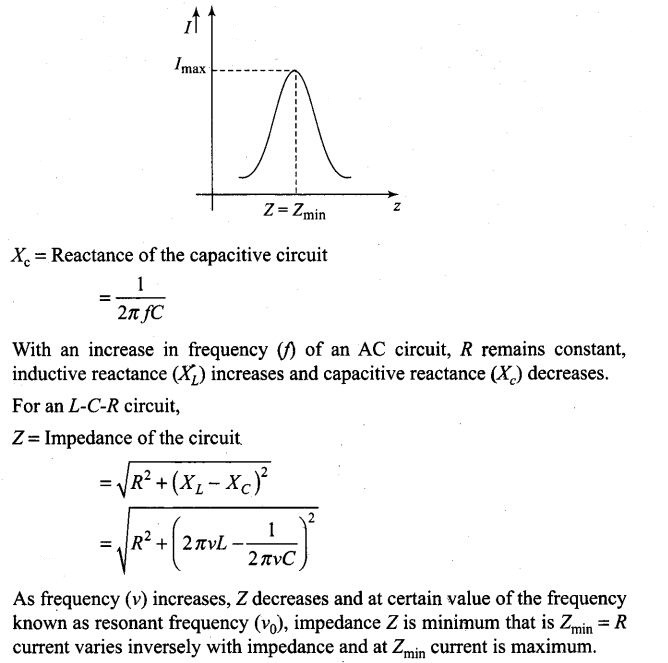Question 9. In an alternating current circuit consisting of elements in series, the current increases on increasing the frequency of supply. Which of the following elements are likely to constitute the circuit?
(a) Only resistor (b) Resistor and an inductor
(c) Resistor and a capacitor (d) Only a capacitor
Solution: (c, d) This is the similar problem as we discussed above. In this problem, the current increases on increasing the frequency of supply. Hence, the reactance of the circuit must be decreased as increase in frequency. So, one element that must be connected is capacitor. We can also connect a resistor in series.
For a capacitive circuit,
XC = 1/ωC =1/2πfC
When frequency increases, XC decreases. Hence current in the circuit increases.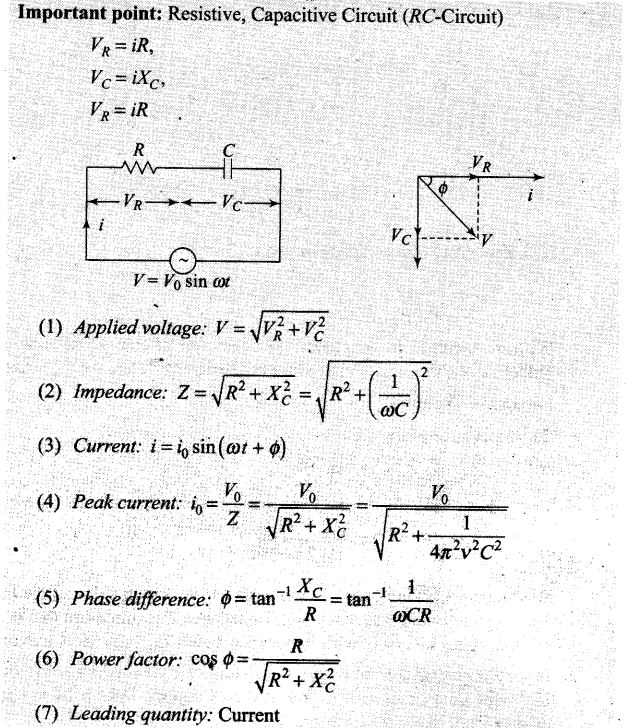Question 10. Electrical energy is transmitted over large distances at high alternating voltages. Which of the following statements is (are) correct?
(a) For a given power level, there is a lower current
(b) Lower current implies less power loss
(c) Transmission lines can be made thinner
(d) It is easy to reduce the voltage at the receiving end using step-down transformers
Solution: (a, b, d)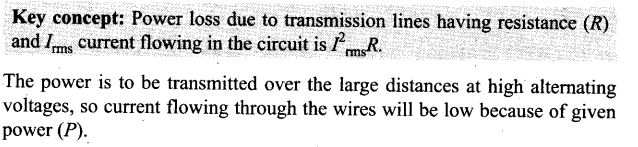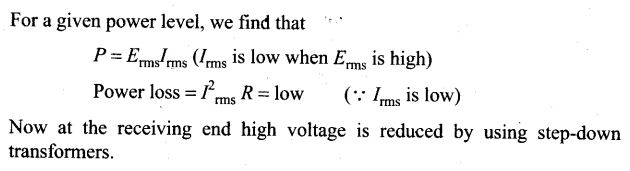Question 11. For an L-C-R circuit, the power transferred from the driving source to the driven oscillator is P = I2 Z cos Ф.
(a) Here, the power factor cos Ф > 0, P > 0
(b) The driving force can give no energy to the oscillator (P = 0) in some cases
(c) The driving force cannot syphon out (P < 0) the energy out of oscillator
(d) The driving force can take away energy out of the oscillator
Solution: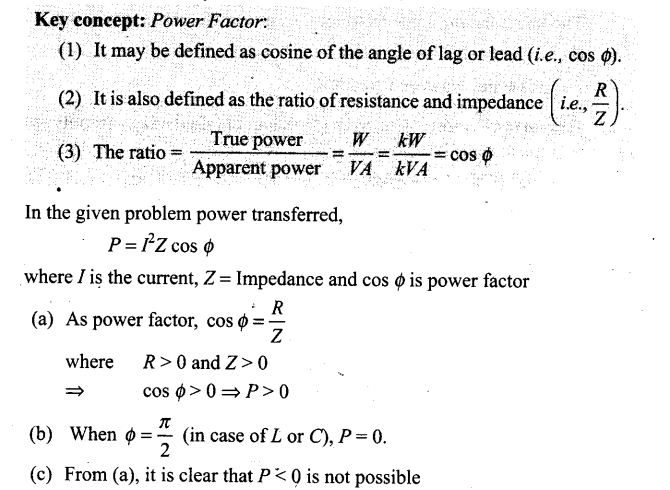Question 12. When an AC voltage of 220 V is applied to the capacitor C
(a) the maximum voltage between plates is 220 V
(b) the current is in phase with the applied voltage
(c) the charge on the plates is in phase with the applied voltage
(d) the power delivered to the capacitor is zero
Solution: (c, d) If the alternating voltage is applied to the capacitor, the plate connected to the positive terminal of the source will be at higher potential and the plate connected to the negative terminal of source will be at lower potential. So the plates capacitor is charged.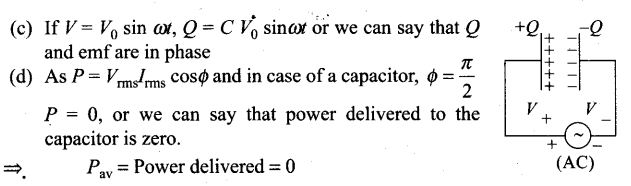Question 13.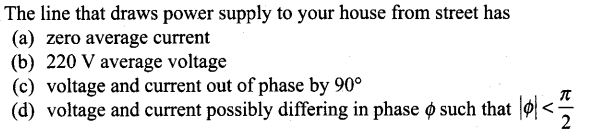Solution: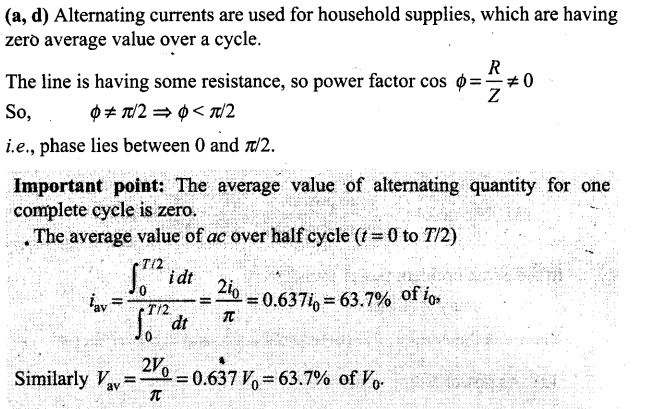Question 14. If an L-C circuit is considered analogous to a harmonically oscillating spring- block system, which energy of the L-C circuit would be analogous to potential energy and which one analogous to kinetic energy?
Solution: When a charged capacitor C having an initial charge q0 is discharged through an inductance L, the charge and current in the circuit start oscillating simple harmonically. If the resistance of the circuit is zero, no energy is dissipated as heat. We also assume an idealized situation in which energy is not radiated away from the circuit. The total energy associated with the circuit is constant.
The oscillation of the LC circuit are an electromagnetic analog to the mechanical oscillation of a block-spring system.
The total energy of the system remains conserved.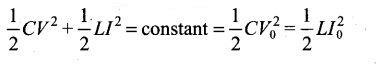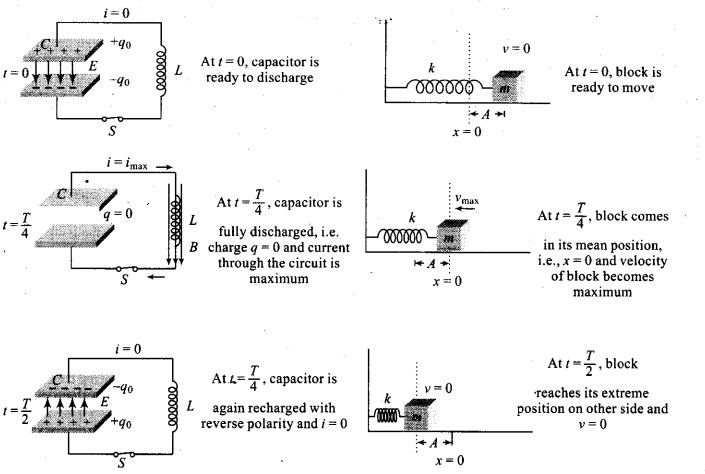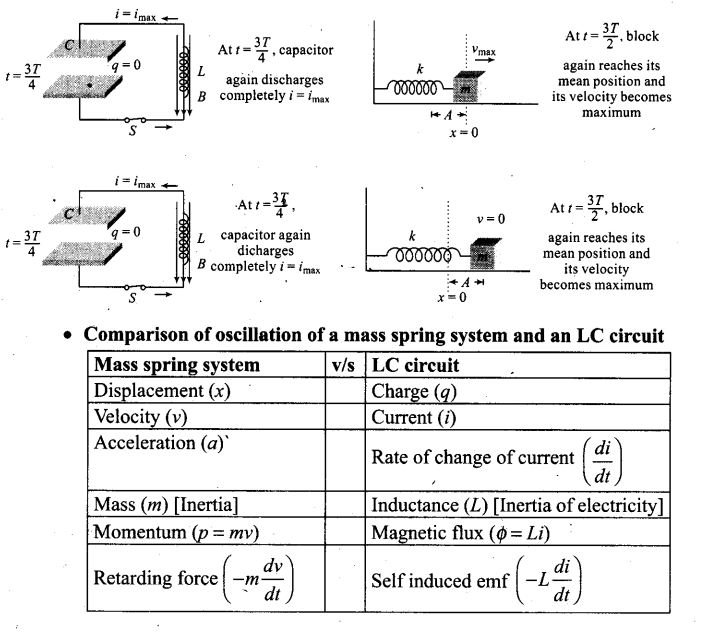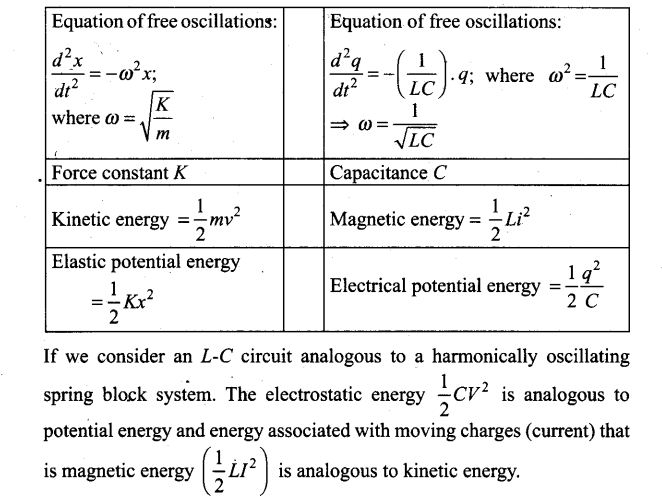Question 15. Draw the effective equivalent circuit of the circuit shown in figure, at very high frequencies and find the effective impedance.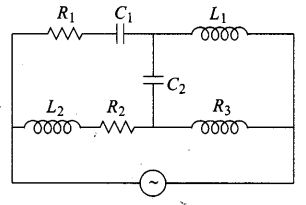Solution:
Key concept: The element with infinite resistance will be considered as open circuit and the element with zero resistance will be considered as short circuited.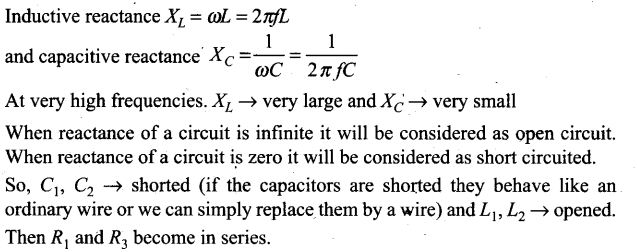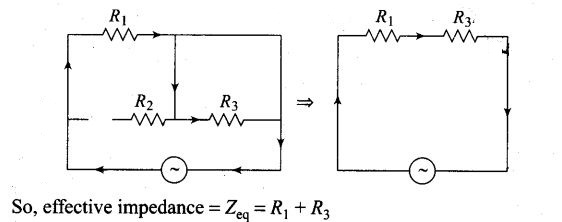Question 16. Study the circuits (a) and (b) shown in figure and answer the following questions.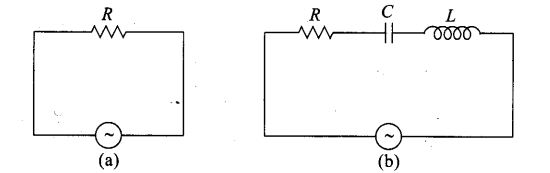(a) Under which conditions would the rms currents in the two circuits be the same?
(b) Can the rms current in circuit (b) be larger than that in (a)?
Solution: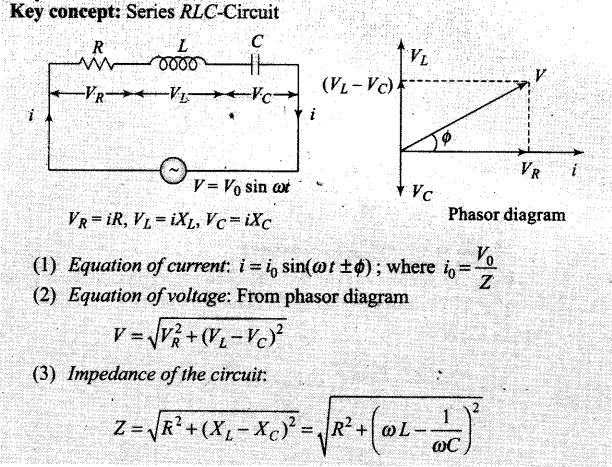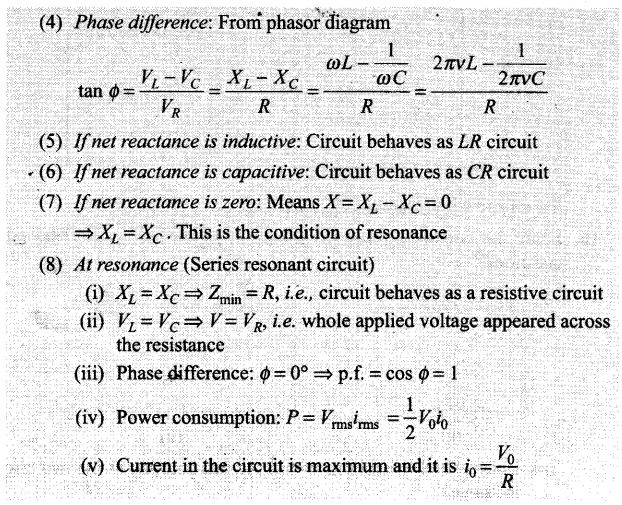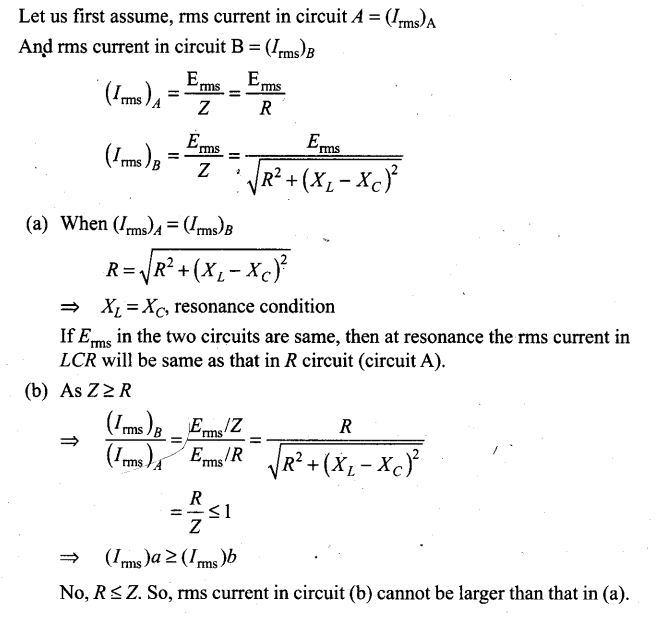Question 17. Can the instantaneous power output of an AC source ever be negative? Can the average power output be negative?
Solution: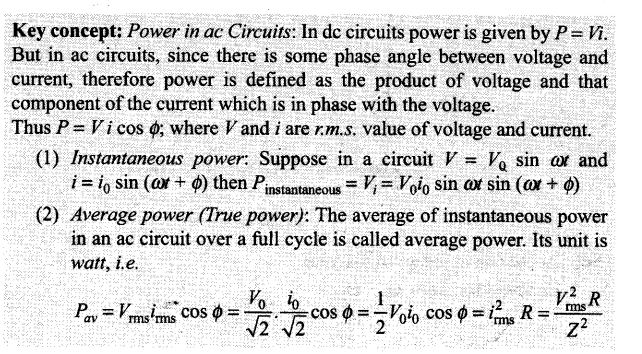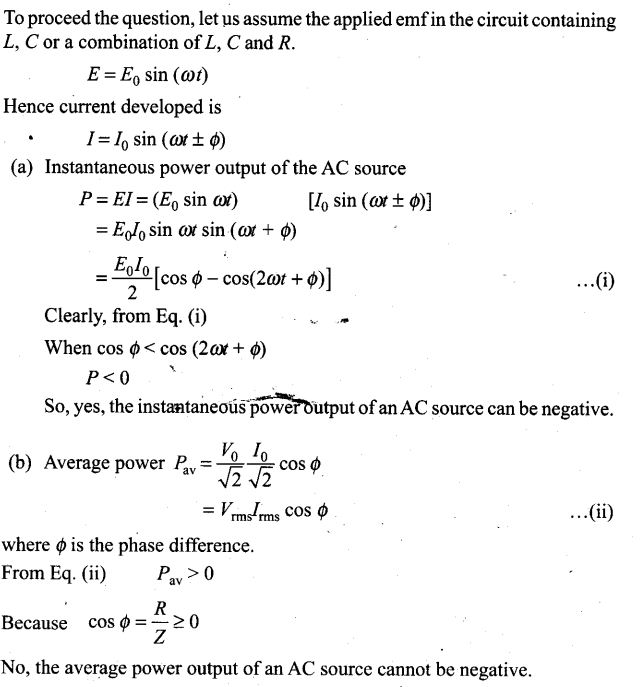Question 18. In a series LCR circuit, the plot of Imax versus co is shown in figure. Find the bandwidth and mark in the figure.Solution: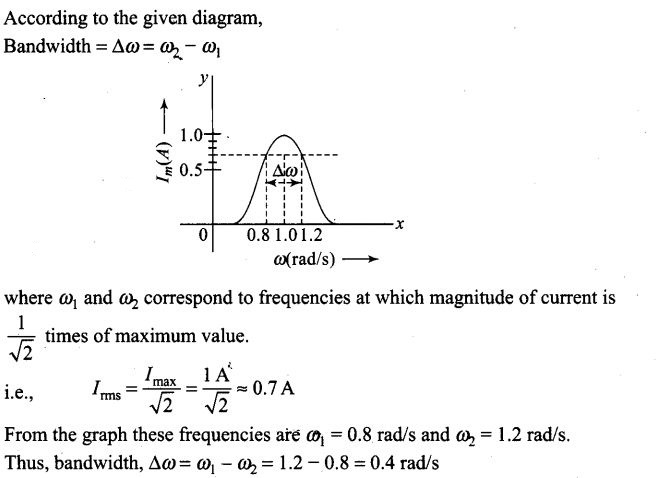Question 19. The alternating current in a circuit is described by the graph shown in figure. Show rms current in this graph.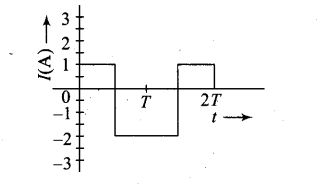Solution: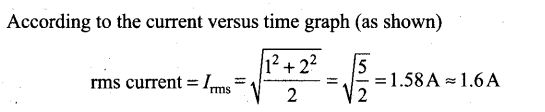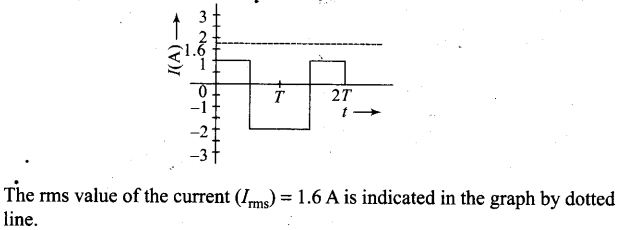Question 20. How does the sign of the phase angle Ф, by which the supply voltage leads the current in an L-C-R series circuit, change as the supply frequency is gradually increased from very low to very high values?
Solution: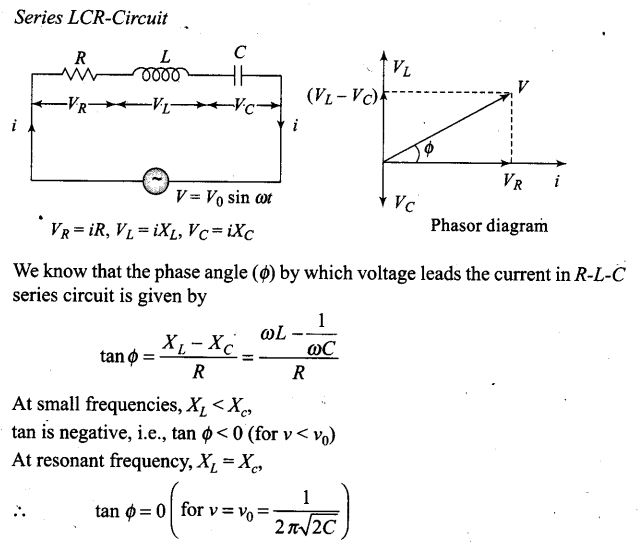Question 21. A device ‘X is connected to an AC source. The variation of voltage, current and power in one complete cycle is shown in figure.
(a) Which curve shows power consumption over a full cycle?
(b) What is the average power consumption over a cycle?
(c) Identify the device X.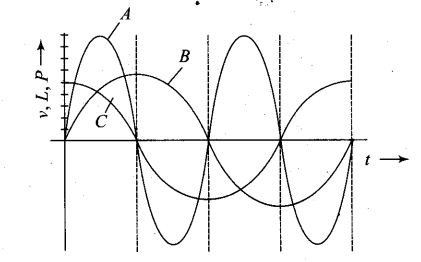Solution: (a) Power is the product of voltage and current (Power = P = VI).
So, the curve of power will be having maximum amplitude, equals to the product of amplitudes of voltage (V) and current (I) curve. Frequencies , of B and C are-equal, therefore they represent V and I curves. So, the curve A represents power.
(b) The full cycle of the graph (as shown by shaded area in the diagram) consists of one positive and one negative symmetrical area.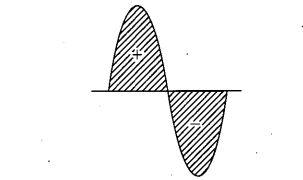Hence, average power consumption over a cycle is zero.
(c) Here phase difference between V and I is π /2 therefore, the device ‘X’ may be an inductor (L) or capacitor (C) or the series combination of L and C.

Question 22. Both alternating current and direct current are measured in amperes. But how is the ampere defined for an alternating current?
Solution: For a Direct Current (DC),
1 ampere = 1 coulomb/sec
Direction of AC changes with the frequency of source with the source frequency and the attractive force would average to zero. Thus, the AC ampere must be defined in terms of some property that is independent of the direction of current. Joule’s heating effect is such property and hence it is used to define rms value of AC.
So, r.m.s. value of AC is equal to that value of DC, which when passed through a resistance for a given time will produce the same amount of heat as produced by the alternating current when passed through the same resistance for same time.

Question 23. A coil of 0.01 H inductance and 1 ω resistance is connected to 200 V, 50 Hz AC supply. Find the impedance of the circuit and time lag between maximum alternating voltage and current.
Solution: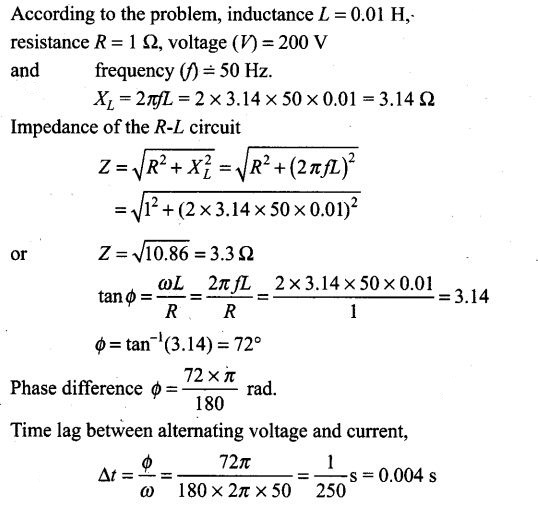Question 24. A 60 W load is connected to the secondary of a transformer whose primary draws line voltage. If a current of 0.54 A flows in the load, what is the current in the primary coil? Comment on the type of transformer being used.
Solution: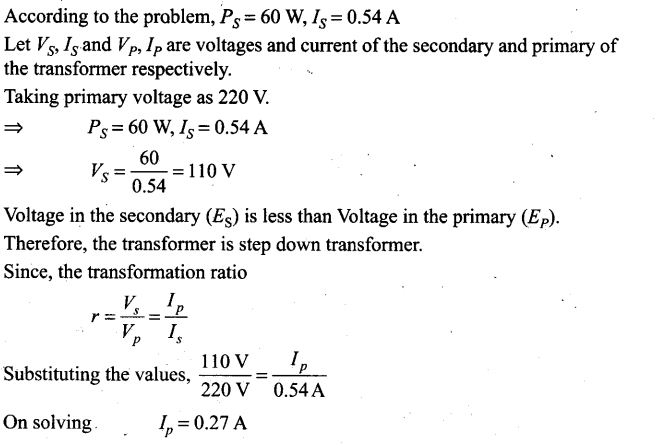Question 25. Explain why the reactance provided by a capacitor to an alternating current decreases with increasing frequency.
Solution: Capacitor plates get charged and discharged when an AC voltage is applied across the plates. So the current through capacitor is as a result of charging charge. Because the frequency of the capacitive circuit increases, the polarities of the charged plates change more rapidly with time, giving rise to a’larger current. The capacitance reactance (XC) due to a capacitor C varies
as the inverse of the frequency (f) (as XC=1/2π fC) and hence approaches zero as v approaches infinity. The current is zero in a DC capacitive circuit, which corresponds to zero proportional and infinite reactance. Also, Since XC is inversely proportional to frequency, capacitors tend to pass high-frequency current and to block low-frequency currents and DC (just the opposite of inductors).

Question 26. Explain why the reactance offered by an inductor increases with increasing frequency of an alternating voltage.
Solution: The inductive reactance is given by XL = 2πfL, XL is proportional to the frequency and current is inversely proportional to the reactance. An inductor opposes the flow of current through it by developing a back emf according to Lenz’s law. If the current is decreasing, the polarity of the induced emf will be so as to increase the current and vice-versa.
Since, the induced emf is proportional to the rate of change of current.

Question 27.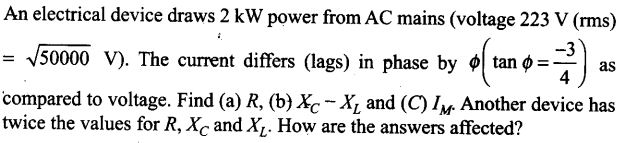Solution: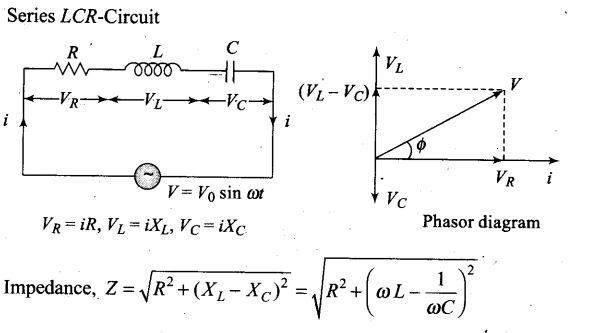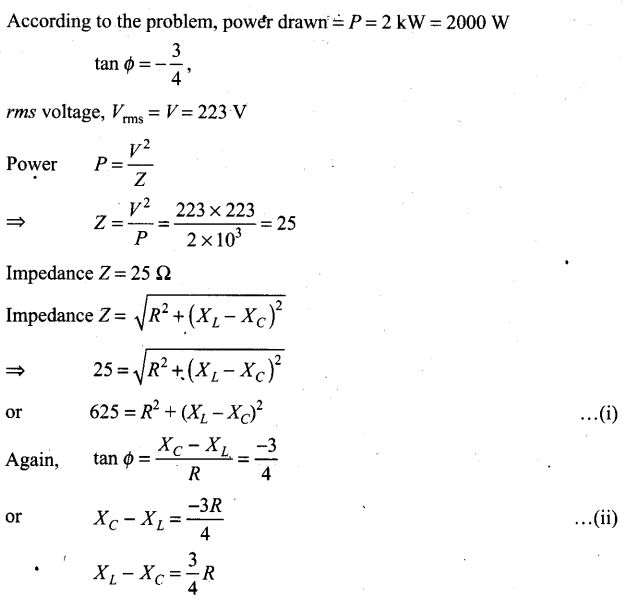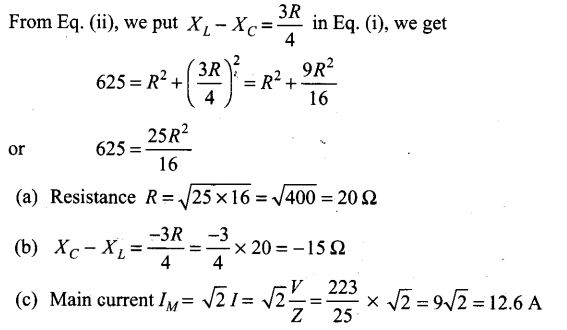Question 28.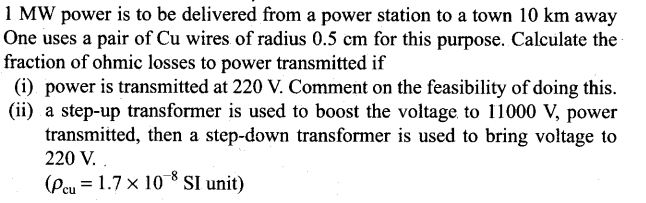Solution: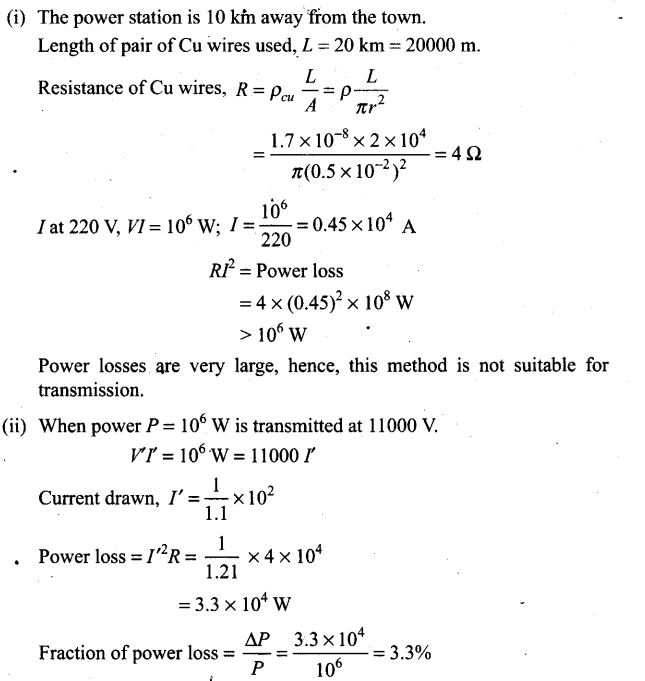Question 29. Consider the L-C-R circuit shown in figure. Find the net current i and the phase of i. Show that i = V/Z. Find the-impedance Z for this circuit.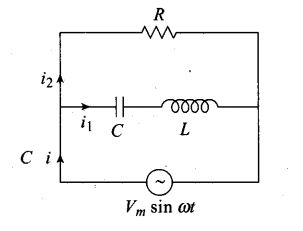Solution: Key concept: In the circuit given above consists of a capacitor (C) and an inductor (L) connected in series and the combination is connected in parallel with a resistance R. Due to this combination there is an oscillation of electromagnetic energy.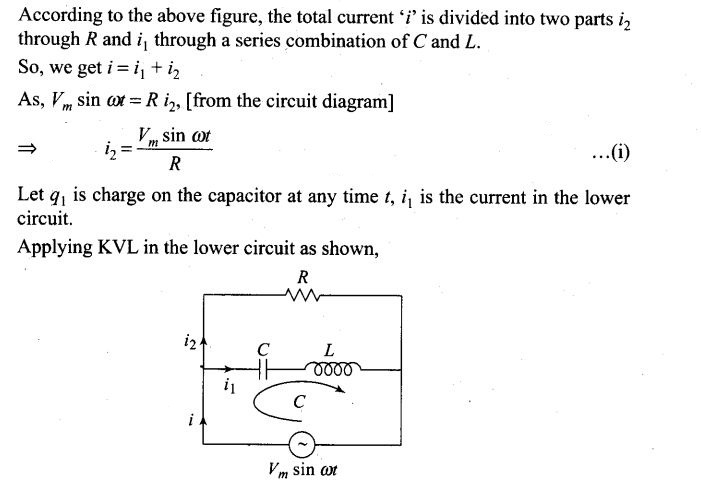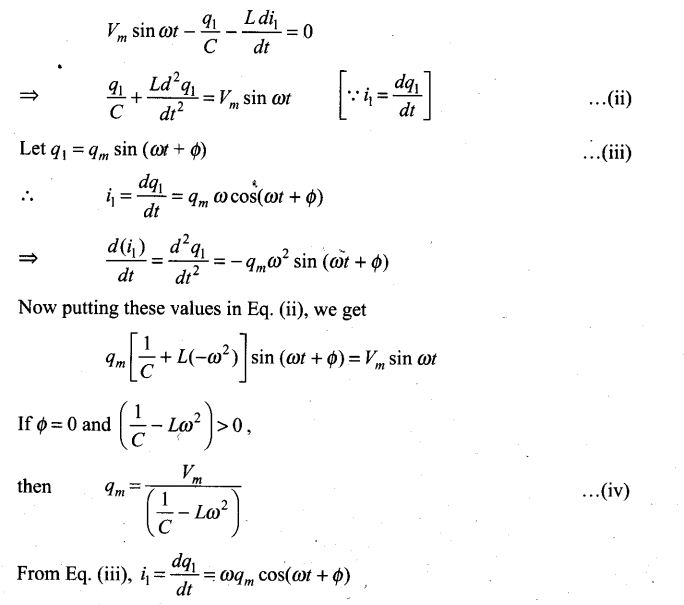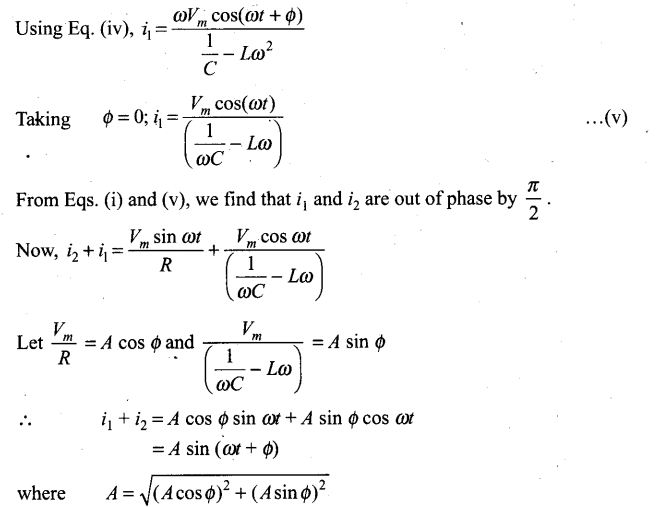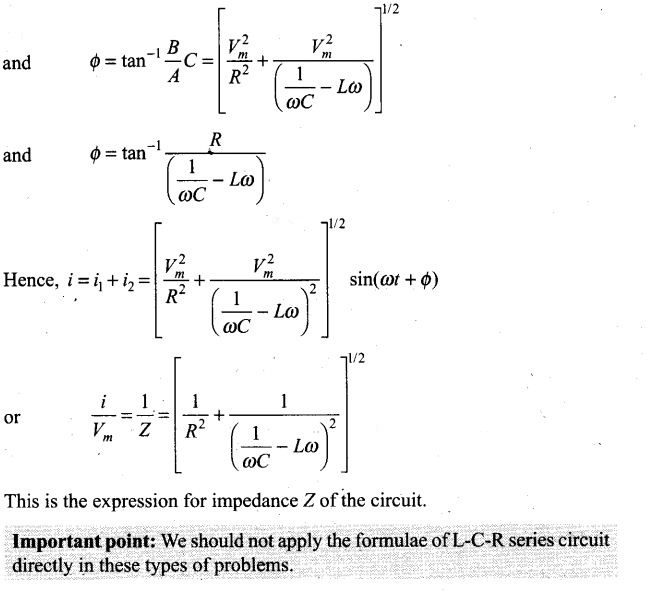Question 30.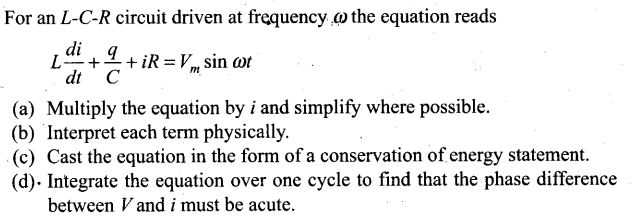Solution: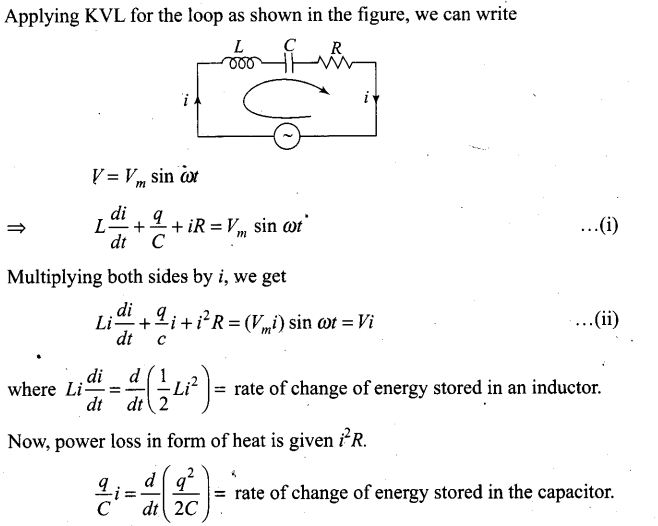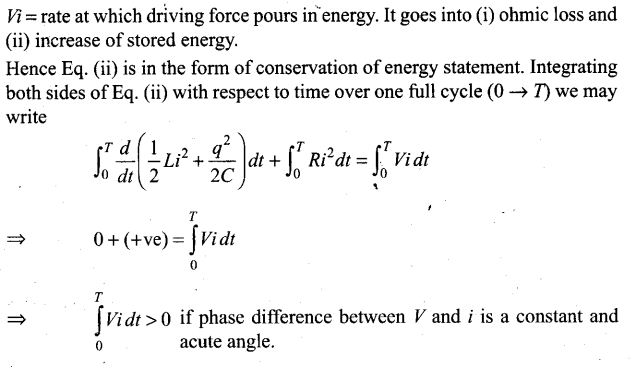Question 31.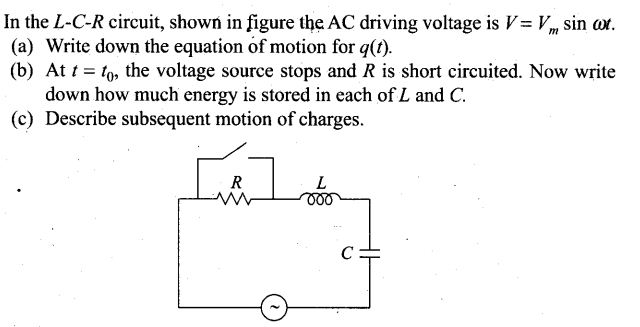Solution: